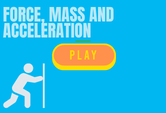# Game Quiz On Force, Mass And Acceleration

This page features a Game Quiz On Force, Mass And Acceleration. It is an interactive quiz with questions covering the three concepts. These princilples are interelated in some ways and are calculated different. Practice and learn with this game that works for classroom and homeschooling purposes. Click and start learning. 5th, 6th, 7th, 8th and 9th grades.N is the SI unit for force. The “N” stands for…

1. Neuton
2. Neutron
3. Nitro
4. Newton

What is net force?

1. The product of all forces acting on an object
2. The sum of all forces acting on an object
3. The quotient of all forces acting on an object
4. The square root of all forces acting on an object

Which of these is an example of force?

1. Pull
2. Push
3. Both A and B
4. Neither

An object’s net force is equal to the ______ of its mass and acceleration

1. Square
2. Product
3. Cube
4. Sum

The above question corresponds to Newton’s ______ Law of Motion

1. First
2. Second
3. Third
4. Zeroth

What is the approximate acceleration of gravity to objects in freefall?

1. 9.8 m/s2
2. 9.8 km/s2
3. 9.8 m/s3
4. 9.8 cm/ms2

Duke pushes a box eastward with a force of 47 N, while Bubba pushes the same box in the opposite direction with a force of 62 N. What is the net force?

1. 109 N
2. -10 N
3. 15 N
4. -109 N

Force has a direction, and is therefore a ______ quantity.

1. Scalar
2. Vector
3. Semi-scalar
4. Non-vector

Colin is dragging along a large log using a rope with a force of 35 N. Holding another rope, his friend Ryan is dragging the same log with a force of 25 N. They are both headed west. What is the net force?

1. 50 N
2. 60 N
3. –50 N
4. -60 N

Which is not a contact force?

1. Tension force
2. Frictional force
3. Applied force
4. Gravitational force

An object needs 30 N of force to accelerate at 1.5 m/sec2. What is its mass?

1. 2 kg
2. 10 kg
3. 20 kg
4. 30 kg

What kind of force is observed when a table exerts an upward force to the downward force of a coffee mug placed on its surface?

1. Tension force
2. Spring force
3. Gravitational force
4. Normal force

What is the acceleration of a 4.2 kg object pushed by a 21 N force?

1. 0.5 m/s2
2. 3.5 m/s2
3. 4.5 m/s2
4. 5 m/s2

Imagine pushing your 38 kg crush forward while ice skating. How much force do you need to exert for them to reach an acceleration of 1.9 m/s2?

1. 72.2 N
2. 7.22 N
3. 722 N
4. 20 N

Which force resists the movement of two surfaces sliding against each other?

1. Frictional force
2. Tension force
3. Normal force
4. Air resistance force